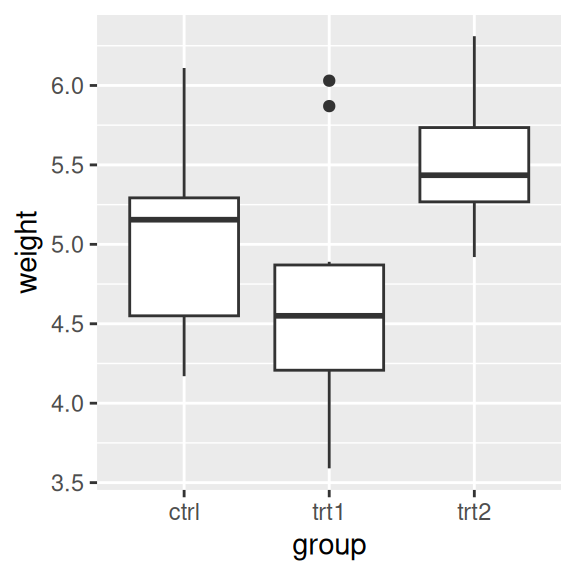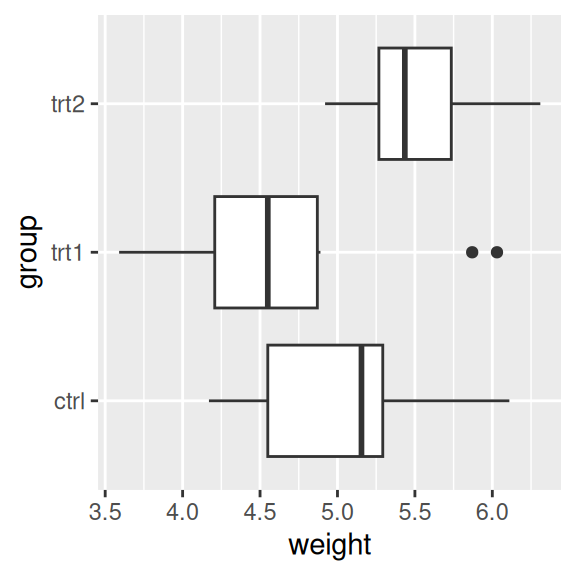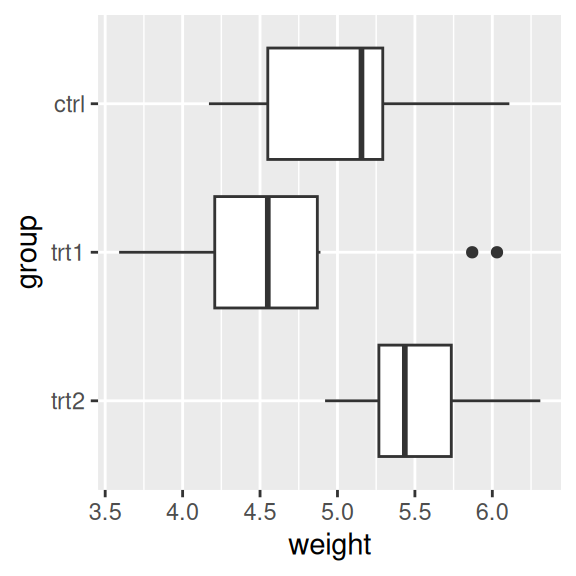## 8.1 Swapping X- and Y-Axes

### 8.1.1 Problem

You want to swap the x- and y-axes on a graph.

### 8.1.2 Solution

Use `coord_flip()` to flip the axes (Figure 8.1):

``````ggplot(PlantGrowth, aes(x = group, y = weight)) +
geom_boxplot()

ggplot(PlantGrowth, aes(x = group, y = weight)) +
geom_boxplot() +
coord_flip()``````Figure 8.1: A box plot with regular axes (left); With swapped axes (right)

### 8.1.3 Discussion

For a scatter plot, it is trivial to change what goes on the vertical axis and what goes on the horizontal axis: just exchange the variables mapped to x and y. But not all the geoms in ggplot treat the x- and y-axes equally. For example, box plots summarize the data along the y-axis, the lines in line graphs move in only one direction along the x-axis, error bars have a single x value and a range of y values, and so on. If you’re using these geoms and want them to behave as though the axes are swapped, `coord_flip()` is what you need.

Sometimes when the axes are swapped, the order of items will be the reverse of what you want. On a graph with standard x- and y-axes, the x items start at the left and go to the right, which corresponds to the normal way of reading, from left to right. When you swap the axes, the items still go from the origin outward, which in this case will be from bottom to top – but this conflicts with the normal way of reading, from top to bottom. Sometimes this is a problem, and sometimes it isn’t. If the x variable is a factor, the order can be reversed by using `scale_x_\$discrete()` with `limits = rev(levels(...))`, as in Figure 8.2:

``````ggplot(PlantGrowth, aes(x = group, y = weight)) +
geom_boxplot() +
coord_flip() +
scale_x_discrete(limits = rev(levels(PlantGrowth\$group)))``````Figure 8.2: A box plot with swapped axes and x-axis order reversed